### Problem Set 4b Financial Planning Class 10th Mathematics Part 1 MHB Solution

Problem Set 4b

1. If the Face Value of a share is Rs. 100 and Market value is Rs. 75,then which of the…
2. What is the amount of dividend received per share of face value Rs. 10 and dividend…
3. The NAV of a unit in mutual fund scheme is Rs. 10.65 then find the amount required to…
4. Rate of GST on brokerage is . . . Write the correct alternative for the following…
5. To find the cost of one share at the time of buying the amount of Brokerage and GST is…
6. Find the purchase price of a share of FV Rs. 100 if it is at premium of Rs. 30. The…
7. Prashant bought 50 shares of FV Rs. 100, having MV Rs. 180. Company gave 40% dividend…
8. Find the amount received when 300 shares of FV Rs. 100, were sold at a discount of Rs.…
9. Find the number of shares received when Rs. 60,000 was invested in the shares of FV Rs.…
10. Smt. Mita Agrawal invested Rs. 10,200 when MV of the share is Rs. 100. She sold 60…
11. Market value of shares and dividend declared by the two companies is given below. Face…
12. Shri. Aditya Sanghavi invested Rs. 50,118 in shares of FV Rs. 100, when the market…
13. Shri. Batliwala sold shares of Rs. 30,350 and purchased shares of Rs. 69,650 in a day.…
14. Smt. Aruna Thakkar purchased 100 shares of FV 100 when the MV is Rs. 1200. She paid…
15. Smt. Anagha Doshi purchased 22 shares of FV Rs. 100 for Market Value of Rs. 660. Find…

###### Problem Set 4b
Question 1.

Write the correct alternative for the following question.

If the Face Value of a share is Rs. 100 and Market value is Rs. 75,then which of the following statements is correct?
A. The share is at premium of Rs. 175

B. The share is at discount of Rs. 25

C. The share is at premium of Rs. 25

D. The share is at discount of Rs. 75

Market value = Rs. 75

Face Value = Rs. 100

If the market value is at a lower price than the face value then the share is in a discount.

Discount = (Face value-Market value) = Rs. 25

(A) The share is at premium Rs. 175 is wrong

(B) The share is at a discount of Rs. 25 is correct

(C) The share is at a premium of Rs. 25 is wrong

(D) The share is at discount of Rs. 75 is wrong

Hence, only B is correct

Question 2.

Write the correct alternative for the following question.

What is the amount of dividend received per share of face value Rs. 10 and dividend declared is 50%.
A. Rs. 50

B. Rs. 5

C. Rs. 500

D. Rs. 100

Rate of dividend = 50%

Dividend =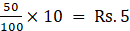Question 3.

Write the correct alternative for the following question.

The NAV of a unit in mutual fund scheme is Rs. 10.65 then find the amount required to buy 500 such units.
A. 5325

B. 5235

C. 532500

D. 53250

Net Asset Value = (N.A.V. of one unit × No. Of units) = Rs. 5325

Question 4.

Write the correct alternative for the following question.

Rate of GST on brokerage is . . .
A. 5%

B. 12%

C. 18%

D. 28%

Rate of GST on Brokerage is 18% according to Central board of Exercise and customs

Question 5.

Write the correct alternative for the following question.

To find the cost of one share at the time of buying the amount of Brokerage and GST is to be . . . the MV of share .

B. substracted from

C. Multiplied with

D. divided by

The Brokerage and GST needs to be added to the Market Value of share at the time of buying.

Question 6.

Find the purchase price of a share of FV Rs. 100 if it is at premium of Rs. 30. The brokerage rate is 0.3%.

Face Value = Rs. 100

Market value = (100 + 30) = Rs. 130

Rate of Brokerage = 0.3%

Brokerage =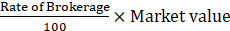Brokerage =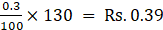Purchase price = (130 + 0.39) = Rs. 130.39

Question 7.

Prashant bought 50 shares of FV Rs. 100, having MV Rs. 180. Company gave 40% dividend on the shares. Find the rate of return on investment.

Face Value = Rs. 100

Market value = Rs. 180

Rate of Dividend = 40%

Dividend =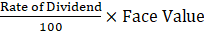⇒ Dividend =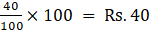No. of shares brought = 50

Total investment = (50 × 180) = Rs. 9000

Total profit = (40 × 50) = Rs. 2000

Rate of Return =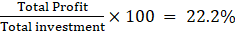Question 8.

Find the amount received when 300 shares of FV Rs. 100, were sold at a discount of Rs. 30.

Face Value = Rs. 100

Discount = Rs. 30

Market Value = (100-30) = Rs. 70

No. Of shares = 300

Amount Received = (70× 300) = Rs. 21,000

Question 9.

Find the number of shares received when Rs. 60,000 was invested in the shares of FV Rs. 100 and MV Rs. 120.

Market Value = Rs. 120

Amount invested = Rs. 60,000

No. of shares received =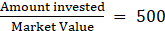Question 10.

Smt. Mita Agrawal invested Rs. 10,200 when MV of the share is Rs. 100. She sold 60 shares when the MV was Rs. 125 and sold remaining shares when the MV was Rs. 90. She paid 0.1% brokerage for each trading. Find whether she made profit or loss? and how much?

Amount Invested = Rs. 10,200

Market value when purchased = Rs. 100

Rate of Brokerage = 0.1%

Brokerage when purchased =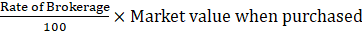Brokerage when purchased =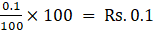Brokerage when sold =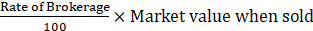Brokerage when sold for first 60 shares =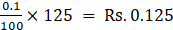Brokerage when remaining shares are sold =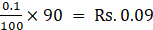No. of shares Purchased =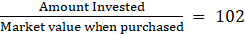No. of shares sold when M.V. is Rs. 125 = 60

No. of shares sold when M.V. is Rs. 90 = (102-60) = 42

Total amount returned = (60× 125) + (42× 90) = Rs. 11,280

Total brokerage paid = (0.1×102) + (0.125×60) + (0.09×42) = Rs. 21.48

Total profit = ( Total amount returned- Amount Invested- Total brokerage paid) = Rs. 1058.52

Question 11.

Market value of shares and dividend declared by the two companies is given below. Face Value is same and it is Rs. 100 for both the shares. Investment in which company is more profitable?

(1) Company A - Rs. 132 , 12%

(2) Company B - Rs. 144, 16%

Face Value = Rs. 100

Market value of Company A share = Rs. 132

Rate of Dividend = 12%

Dividend =⇒ Dividend of Company A share =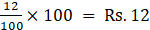Market value of Company B share = Rs. 144

Rate of Dividend of Company B share = 16%

Dividend =⇒ Dividend =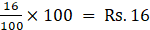Return% of Company A =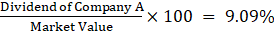Return% of Company B =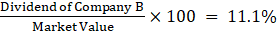Return % of Company B is more so company B is more profitable.

Question 12.

Shri. Aditya Sanghavi invested Rs. 50,118 in shares of FV Rs. 100, when the market value is Rs. 50. Rate of brokerage is 0.2% and Rate of GST on brokerage is 18%, then How many shares were purchased for Rs. 50,118?

Amount invested = Rs. 50,118

Let number of shares be n

Total Share Price = Market Value × Number of Shares = 50 n

Now Brokerage is calculated over total share price, Brokerage = 0.2% of 50 n

G.S.T is calculated over brokerage money, G.S.T = 18% of 0.2% of 50 n

Therefore,

(Total Share Price + Brokerage + G.S.T) = Money Invested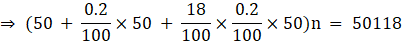(50 n + 0.1 n + 0.018 n) = 50118

⇒ 50.118 n = 50118

⇒ n = 1000 shares

Hence, The total number of shares are 1000.

Question 13.

Shri. Batliwala sold shares of Rs. 30,350 and purchased shares of Rs. 69,650 in a day. He paid brokerage at the rate of 0.1% on sale and purchase. 18% GST was charged on brokerage. Find his total expenditure on brokerage and tax.

Selling Price = Rs. 30,350

Rate of brokerage = 0.1%

Brokerage when sold =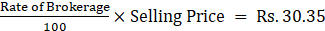Purchase price = Rs. 69,650

Brokerage when purchased =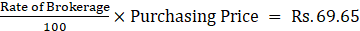Total Brokerage = Rs.100

Rate of GST = 18%

Total GST =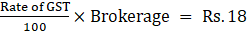Total expenditure on Brokerage and GST = (100 + 18) = Rs. 118

Question 14.

Smt. Aruna Thakkar purchased 100 shares of FV 100 when the MV is Rs. 1200. She paid brokerage at the rate of 0.3% and 18% GST on brokerage.

Find the following -

(1) Net amount paid for 100 shares.

(2) Brokerage paid on sum invested.

(3) GST paid on brokerage.

(4) Total amount paid for 100 shares.

No. of shares = 100

Market value = Rs. 1200

(1) Net amount paid = (100× 1200) = Rs. 1,20,000

Rate of Brokerage = 0.3%

(2) Brokerage paid =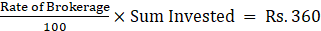Rate of GST = 18%

(3) Total GST =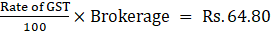(4) Total amount paid for 100 shares = (120000 + 360 + 64.80) = Rs. 1,20,424.80

Question 15.

Smt. Anagha Doshi purchased 22 shares of FV Rs. 100 for Market Value of Rs. 660. Find the sum invested. After taking 20% dividend, she sold all the shares when market value was Rs. 650. She paid 0.1% brokerage for each trading done. Find the percent of profit or loss in the share trading. (Write your answer to the nearest integer.)

No. of shares = 22

Face Value = Rs. 100

Market Value when purchased = Rs. 660

Market Value when sold = Rs. 650

Rate of brokerage = 0.1 %

Brokerage paid when purchased =Brokerage paid when purchased =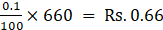Brokerage paid when sold =Brokerage paid when sold =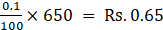Total value of purchase = (660 + 0.66)× 22 = Rs. 14534.52

Total value of sell = (650-0.66)× 22 = Rs. 14285.48

Loss in share selling = ( Total value of purchase- Total value of sell) = Rs. 249.04

Rate of Dividend = 20%

Dividend =⇒ Dividend =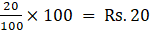Total dividend = (20× 22) = Rs. 440

Overall profit in the trade = ( Total dividend- Loss in share selling) = Rs. 190.96

Profit percent =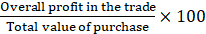⇒ Profit percent =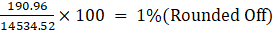## PDF FILE TO YOUR EMAIL IMMEDIATELY PURCHASE NOTES & PAPER SOLUTION. @ Rs. 50/- each (GST extra)

SUBJECTS

HINDI ENTIRE PAPER SOLUTION

MARATHI PAPER SOLUTION
SSC MATHS I PAPER SOLUTION
SSC MATHS II PAPER SOLUTION
SSC SCIENCE I PAPER SOLUTION
SSC SCIENCE II PAPER SOLUTION
SSC ENGLISH PAPER SOLUTION
SSC & HSC ENGLISH WRITING SKILL
HSC ACCOUNTS NOTES
HSC OCM NOTES
HSC ECONOMICS NOTES
HSC SECRETARIAL PRACTICE NOTES

2019 Board Paper Solution

HSC ENGLISH SET A 2019 21st February, 2019

HSC ENGLISH SET B 2019 21st February, 2019

HSC ENGLISH SET C 2019 21st February, 2019

HSC ENGLISH SET D 2019 21st February, 2019

SECRETARIAL PRACTICE (S.P) 2019 25th February, 2019

HSC XII PHYSICS 2019 25th February, 2019

CHEMISTRY XII HSC SOLUTION 27th, February, 2019

OCM PAPER SOLUTION 2019 27th, February, 2019

HSC MATHS PAPER SOLUTION COMMERCE, 2nd March, 2019

HSC MATHS PAPER SOLUTION SCIENCE 2nd, March, 2019

SSC ENGLISH STD 10 5TH MARCH, 2019.

HSC XII ACCOUNTS 2019 6th March, 2019

HSC XII BIOLOGY 2019 6TH March, 2019

HSC XII ECONOMICS 9Th March 2019

SSC Maths I March 2019 Solution 10th Standard11th, March, 2019

SSC MATHS II MARCH 2019 SOLUTION 10TH STD.13th March, 2019

SSC SCIENCE I MARCH 2019 SOLUTION 10TH STD. 15th March, 2019.

SSC SCIENCE II MARCH 2019 SOLUTION 10TH STD. 18th March, 2019.

SSC SOCIAL SCIENCE I MARCH 2019 SOLUTION20th March, 2019

SSC SOCIAL SCIENCE II MARCH 2019 SOLUTION, 22nd March, 2019

XII CBSE - BOARD - MARCH - 2019 ENGLISH - QP + SOLUTIONS, 2nd March, 2019

HSC Maharashtra Board Papers 2020

(Std 12th English Medium)

HSC ECONOMICS MARCH 2020

HSC OCM MARCH 2020

HSC ACCOUNTS MARCH 2020

HSC S.P. MARCH 2020

HSC ENGLISH MARCH 2020

HSC HINDI MARCH 2020

HSC MARATHI MARCH 2020

HSC MATHS MARCH 2020

SSC Maharashtra Board Papers 2020

(Std 10th English Medium)

English MARCH 2020

HindI MARCH 2020

Hindi (Composite) MARCH 2020

Marathi MARCH 2020

Mathematics (Paper 1) MARCH 2020

Mathematics (Paper 2) MARCH 2020

Sanskrit MARCH 2020

Important-formula

THANKS